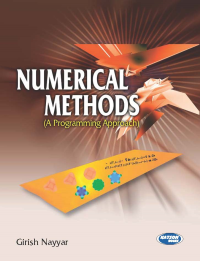•## Numerical Methods

In Stock
ISBN 978-93-80027-96-8 Girish Nayyar S.K. Kataria & Sons 2009 Print In Stock
Rs 395.00Rs 356.00

#### CONTENTS

• Number System and Error Analysis • Algebraic and Transcendental Equations • Simultaneous Linear Algebraic Equations • Matrix Inversion and Eigne Values • Curve Fitting and Method of Least Squares • Finite Differences • Interpolation • Numerical Differentiation • Numerical Integration • Ordinary Differential Equation • Numerical Solution of Partial Differential Equations • Bibliography.

• Author : Girish Nayyar
• Publisher : S.K. Kataria & Sons
• Publishing Year : 2009